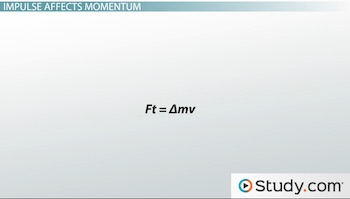# Momentum and Impulse: Definition, Theorem and Examples

An error occurred trying to load this video.

Try refreshing the page, or contact customer support.

Coming up next: Conservation of Linear Momentum: Formula and Examples

### You're on a roll. Keep up the good work!

Replay
Your next lesson will play in 10 seconds
• 0:03 Impulse Affects Momentum
• 2:30 Changing Time and Force
• 4:46 Lesson Summary
Save Save

Want to watch this again later?

Timeline
Autoplay
Autoplay
Speed Speed Audio mode

#### Recommended Lessons and Courses for You

Lesson Transcript
Instructor: Sarah Friedl

Sarah has two Master's, one in Zoology and one in GIS, a Bachelor's in Biology, and has taught college level Physical Science and Biology.

To understand how a change in momentum affects an object, we look to impulse. In this lesson, you'll understand how impulse describes an object's change in momentum, as well as how changing the force or time of the impulse can have very different outcomes.

## Impulse Affects Momentum

Any moving object can have momentum. This is because momentum is mass in motion. The way we determine an object's momentum is fairly straightforward. Momentum is the object's mass times its velocity, or, in equation form, p=mv, where p is momentum, m is mass in kilograms, and v is velocity in meters per second. Momentum is proportional to both mass and velocity, meaning that a change in one will cause the same amount of change in the other. So if you increase an object's mass, you also increase its momentum. The same is true for velocity: increase or decrease the object's speed, and you increase or decrease its momentum by the same amount.

But usually it's the object's velocity that changes instead of its mass, right? You may remember that a change in velocity means the object is accelerating. You may also remember that acceleration is caused by a force and that the greater the force, the greater the acceleration. Therefore, the greater the acceleration, the greater the momentum!

Force is an important factor, but time also counts. Specifically, when we are interested in knowing how long the force acts. For example, if you push a box across the floor for just a few seconds, the time interval is very short. But if you push a box across the floor and you do so with the same force as before, but this time for several minutes, you've increased the amount of time the force acts. This longer time interval leads to a greater change in momentum. This change in momentum is called impulse, and it describes the quantity that we just saw: the force times the time interval it acts over. The greater the impulse, the greater the change in momentum. To change the impulse, you can either change the amount of force, or you can change the time interval in which that force acts. In equation form, we can write this relationship between impulse and momentum as:The Greek letter delta means 'change in,' and we read this equation as force times the time interval equals change in mass times velocity. Be careful not to read this as 'force times time equals mass times velocity' because now you're saying that 'impulse equals momentum.' It's important to remember that impulse is a change in momentum, not momentum itself.

## Changing Time and Force

If you want a large increase in momentum, you need to exert a large force over a long time period. When you quickly shove the box, it doesn't move very quickly across the floor, but if you push it with that same force for a long period of time, you can move it all the way across the room. This is because you've increased the time variable on the left side of the equation. When an object is brought to rest, its momentum also changes, but now it decreases instead of increases. Time is especially important in this situation because a longer time interval means less force acts on the object to result in the same change in momentum.

Say for example that a stunt double jumped from a 10-story window during an action scene. Does she want to land on the sidewalk, which is very hard, or does she want to land on a soft cushion? She definitely wants to land on the soft cushion because this cushioning will increase the time of her impact and decrease the force on her as she lands. In either case, her impulse, or her change in momentum, is exactly the same because in either case, she goes from moving to a state of rest. The difference is that the change occurs over a different time period and with a different amount of force.

To unlock this lesson you must be a Study.com Member.

### Register to view this lesson

Are you a student or a teacher?

#### See for yourself why 30 million people use Study.com

##### Become a Study.com member and start learning now.
Back
What teachers are saying about Study.com

### Earning College Credit

Did you know… We have over 200 college courses that prepare you to earn credit by exam that is accepted by over 1,500 colleges and universities. You can test out of the first two years of college and save thousands off your degree. Anyone can earn credit-by-exam regardless of age or education level.